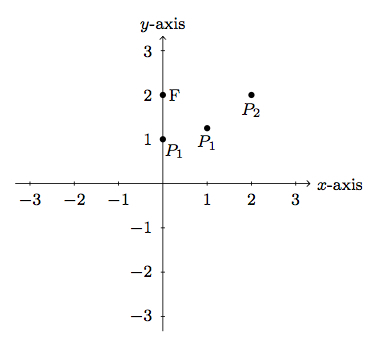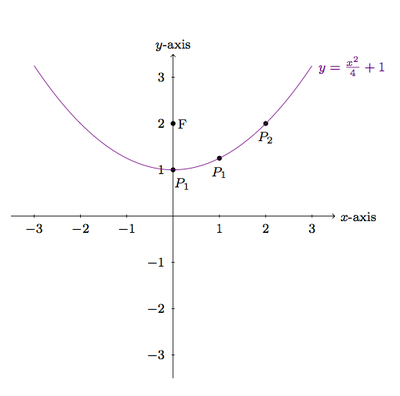# Defining Parabolas Geometrically

Alignments to Content Standards: G-GPE.A.2

Suppose $F = (0,2)$ and $\ell$ is the $x$-axis:1. Find the point on the $y$-axis equidistant from $F$ and $\ell$. Label this point $P_0$.
2. Find the point on the line $x=1$ which is equidistant from $F$ and $\ell$. Label this point $P_1$.
3. Find the point on the line $x = 2$ which is equidistant from $F$ and $\ell$. Label this point $P_2$.
4. If this process is repeated for the vertical lines $x = a$ for all real numbers $a$, what curve do the points $P_a$ make?

## IM Commentary

The purpose of this task is to show, in a particular case, that the points on a parabola can be characterized by being equidistant from a point (the focus) and a line (the directrix). In this case, the point is $F = (0,2)$ and the line is the $x$-axis. The choice of a horizontal line for the directrix is important as this produces a parabola defined by an equation of the form $y = ax^2 + bx + c$. If geometry software is available, students can vary the focus and directrix and see how the corresponding parabola moves. This can also be seen algebraically by changing the coordinates of $F$ and/or moving the directrix. It would be a very interesting and challenging exercise to study how the focus and directrix change when the parabola is translated and/or stretched.

Some guidance may be necessary to help students with parts (a) through (c). The $x$-coordinate is given in these cases so, in part (b) for example, the goal is to find the number $y$ so that the distance from $(1,y)$ to $F$ is the same as the distance from $(1,y)$ to the $x$-axis. If needed, the teacher may also suggest to use the Pythagorean theorem.

Students progressing this task will have ample opportunity to express SMP 8, making use of repeated reasoning as they repeat and then generalize the process of finding the point in question.

## Solution

The points $P_0$, $P_1$, and $P_2$ are shown and labeled here with the calculations given below:1. The point equidistant from $F$ and the $y$-axis is $P_0 = (0,1)$, the bisector of the segment joining $(0,0)$ and $(0,2)$.
2. To find the point $P_1$ equidistant from $F$ and the line $x = 1$, we know that $P_1 = (1,y)$ for some number $y$. The distance from $(1,y)$ to $\ell$ is $y$ while its distance from $F$ can be found using the Pythagorean Theorem: $$\sqrt{1+(y-2)^2}.$$ So we need to solve the equation $$y = \sqrt{1+ (y-2)^2}.$$ Squaring both sides gives $y^2 = 1 + (y-2)^2$ or $-4y + 5 = 0$. So $y = \frac{5}{4}$. This means that $P_1 = \left(1, \frac{5}{4}\right)$.
3. To find the point $P_2$ equidistant from $F$ and the line $x = 2$, we know that $P_2 = (2,y)$ for some number $y$. The distance from $(2,y)$ to $\ell$ is $y$ while its distance from $F$ can be found using the Pythagorean Theorem: $$\sqrt{4+(y-2)^2}.$$ So we need to solve the equation $$y = \sqrt{4+ (y-2)^2}.$$ Squaring both sides gives $y^2 = 4 + (y-2)^2$ or $-4y + 8 = 0$. So $y = 2$ and $P_2 = \left(2, 2\right).$ In this case, we can readily see that $P_2$ is a distance of 2 from both $F$ and $\ell$.
4. We can try this procedure with $y = a$. The distance from $(a,y)$ to $\ell$ is $y$ while its distance from $F$ is $$\sqrt{a^2 + (y-2)^2}$$ by the Pythagorean Theorem. Setting these two quantities equal and squaring both sides gives $$y^2 = a^2 + (y-2)^2.$$ After simplifying we find $-4y + a^2 + 4 = 0$ or $y = \frac{a^2 + 4}{4}$. This means that $P_a = \left(a,\frac{a^2+4}{4}\right)$. The set of all of these points is the graph of the function $y = \frac{x^2}{4} + 1$ which agrees with our calculations in parts a, b, and c above. The graph of this parabola, along with the plotted points, is shown here: